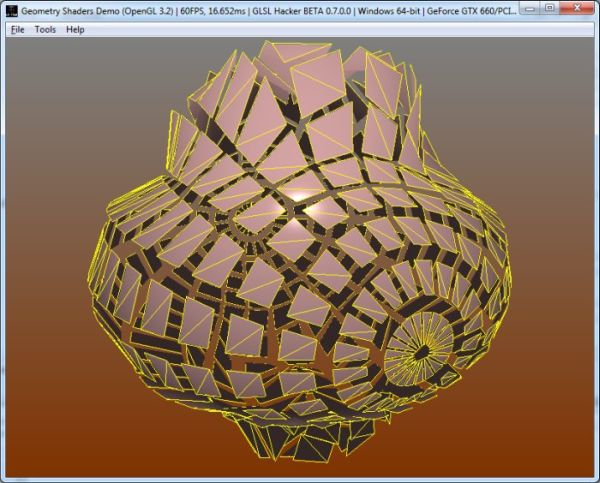# Mesh Exploder with Geometry ShadersToday, a simple but cool geometry shader (OpenGL 3.2) based demo: a mesh exploder. This demo is a simple use-case of geometry shaders where geometry shaders do not create (amplification) or kill vertices. They just deform input vertices. If you need some explanations about GS, you can read these articles:

 The demo of this article has been coded with GLSL Hacker (Windows, OS X and Linux) and you can find the source code (Lua + GLSL) in the Code Sample Pack in the host_api/gl-320-arb-geometry-shader_Mesh_Exploder/ folder. As usual, the GLSL program of this article is not specific to GLSL Hacker. You can use it in any OpenGL/WebGL application with minor changes only (shader inputs like transformation matrices).

## The Geometry Exploder

The principle of the exploder is quite simple: vertices are pushed along a normal vector in the geometry shader. The normal in question is the normal vector of the input face (here a triangle) instead of the regular vertex normal. The demo shows how to compute the normal of a triangle in the geometry shader. Using the normal face, we get this explode effect as if each face of the mesh was separated. The amplitude of the deformation is based on the elapsed time.

The original mesh is a simple sphere. The mesh sphere is rendered twice: the first time in solid mode with a Phong lighting, the second time in wireframe mode (yellow lines).The exploded sphere

Here is a bad quality preview:

Here is the GLSL program (named color_exploder_prog in the demo) used to explode the mesh in the wireframe pass:

```#version 330
in vec4 gxl3d_Position;
in vec4 gxl3d_Normal;

out Vertex
{
vec4 normal;
vec4 color;
} vertex;

void main()
{
gl_Position = gxl3d_Position;
vertex.normal = gxl3d_Normal;
vertex.color =  vec4(1.0, 1.0, 0.0, 1.0);
}
```

```#version 150
layout(triangles) in;
layout(triangle_strip, max_vertices=3) out;

uniform float normal_length;
uniform float time;

// GLSL Hacker automatic uniform
uniform mat4 gxl3d_ModelViewProjectionMatrix;

in Vertex
{
vec4 normal;
vec4 color;
} vertex[];

out vec4 vertex_color;

void main()
{
//------ Face normal
//
vec3 P0 = gl_in.gl_Position.xyz;
vec3 P1 = gl_in.gl_Position.xyz;
vec3 P2 = gl_in.gl_Position.xyz;

vec3 V0 = P0 - P1;
vec3 V1 = P2 - P1;

// If the diff between V0 and V1 is too small,
// the normal will be incorrect as well as the deformation.
//
vec3 diff = V1 - V0;
float diff_len = length(diff);

vec3 N = normalize(cross(V1, V0));

//------ Generate a new face along the direction of the face normal
// only if diff_len is not too small.
//
if (length(diff_len) > 0.001)
{
int i;
for(i=0; i<gl_in.length(); i++)
{
vec4 P = gl_in[i].gl_Position;
vec3 N = normalize(cross(V1, V0));
float len = sqrt(P.x*P.x + P.z*P.z);
float scale = 2.0 + 1.0 * cos(time*2.0 + len);
P = vec4(P.xyz + (N * normal_length * scale) + \
(N * vec3(0.05, 0.05, 0.05)), 1.0);
gl_Position = gxl3d_ModelViewProjectionMatrix * P;
vertex_color = vertex[i].color;
EmitVertex();
}
EndPrimitive();
}
}
```

```#version 150
in vec4 vertex_color;
out vec4 FragColor;
void main()
{
FragColor = vertex_color;
}
```

## 2 thoughts on “Mesh Exploder with Geometry Shaders”

1.erion

Nice!